``` Author : Artyom Martynenko Category : Indicators Last Update : 2 August 2013 Rent Price : 10 USD Version : 1.1 ```

### Description:

The indicator draws the colored weighted moving geometrical mean in the price chart window. Besides, this indicator can be used for colored drawing of other indicators that do not have this feature as a default one. “Trend state Geometrical Mean” can be drawn using other indicator values but in this case, all values of this indicator should be greater than zero.

### Parameters:

• Averaging period – indicator averaging period.

• First weight – weight of the first (earliest) averaging period values.
• Weight incrementation – incrementation of the averaged values’ weight.
• Vertical shift – vertical shift of the indicator in points.
• Horizontal shift – horizontal shift of the indicator in bars.
“Averaging period” parameter should be greater than zero. In addition, the following ratio between parameter values should be true:

(FW * 2) != (WI * (1 – AP)) where:

• FW – “First weight” parameter value.

• WI – “Weight incrementation” parameter value.
• AP – “Averaging period” parameter value.
• “!=” – “Not equal” ratio.
No limits are imposed on “Vertical shift” and “Horizontal shift” parameter values.

### Examples of Settings:

• In order to draw standard moving geometrical mean, set “First weight” parameter to one, while “Weight incrementation” should be equal to zero.

md5-e250cf42daac272fd2cc54bd1d5f6688

### Image of Trend state Geometrical Mean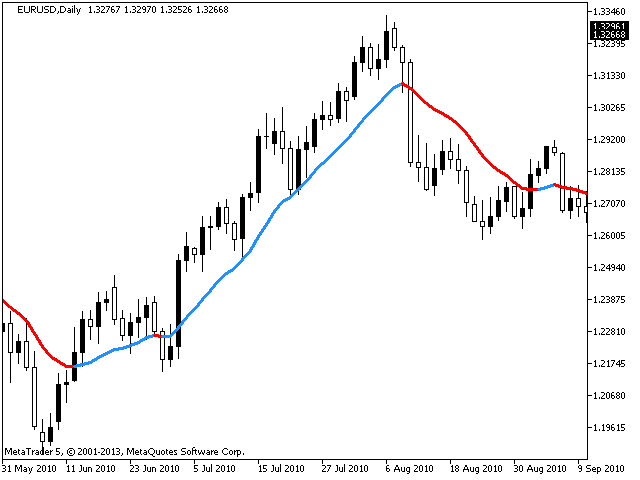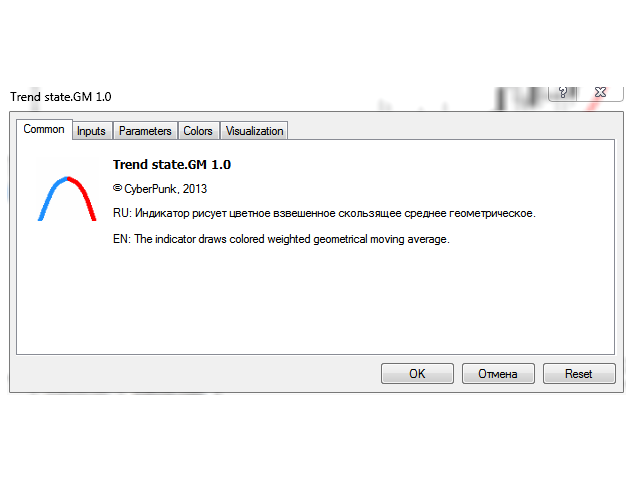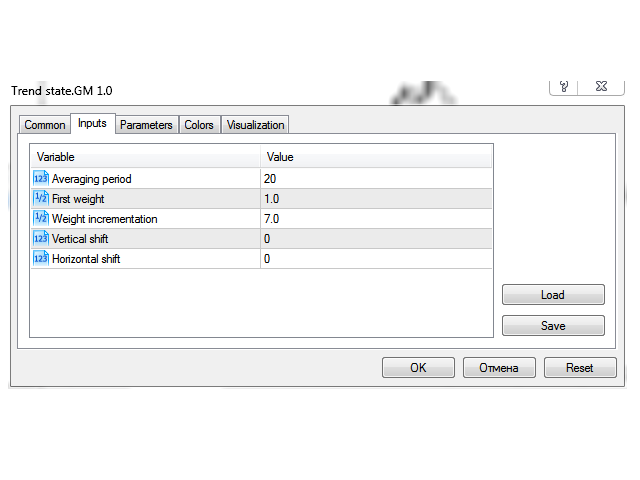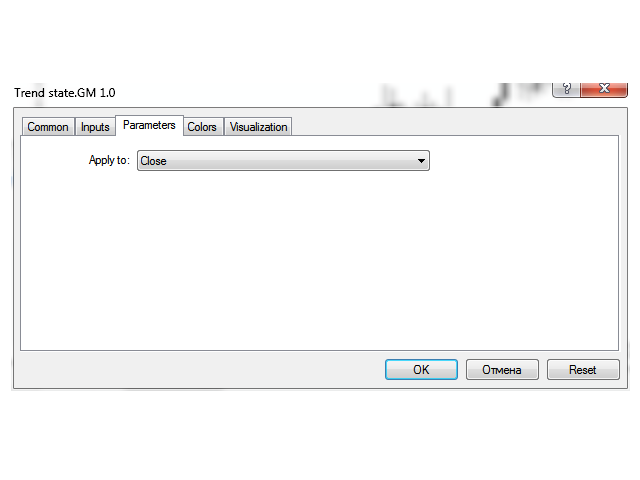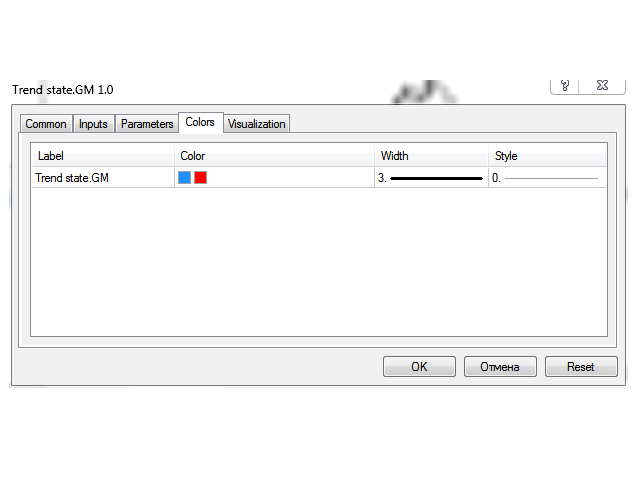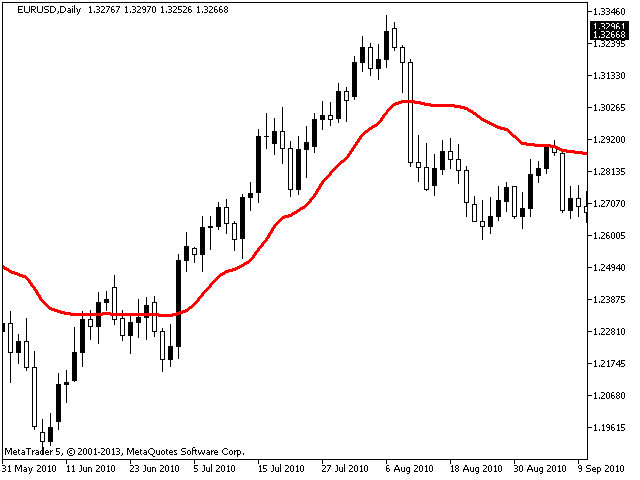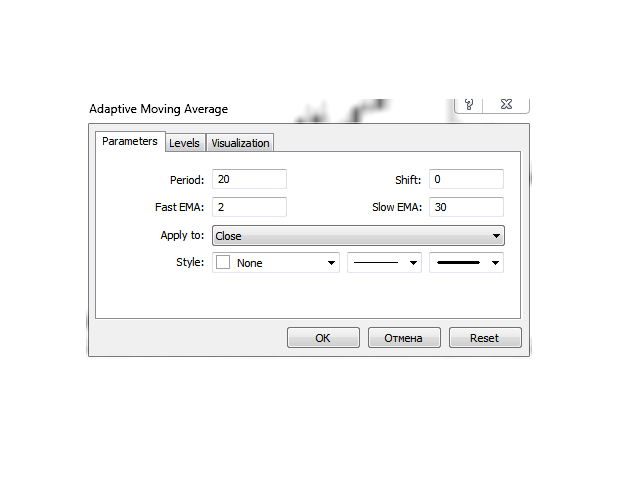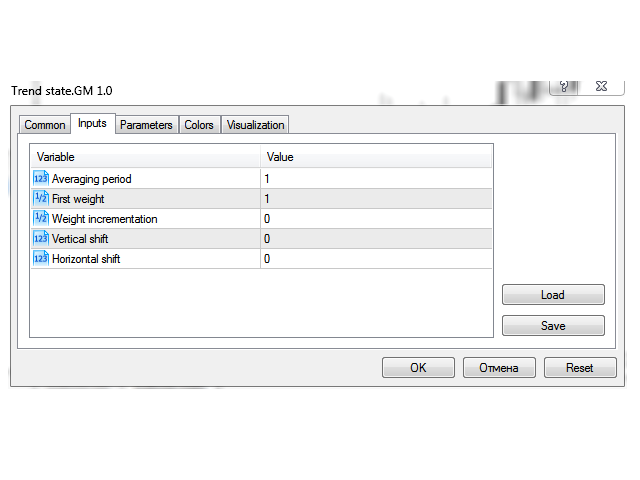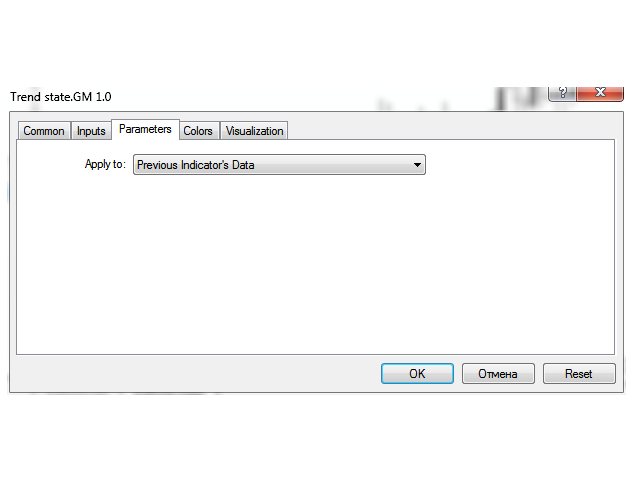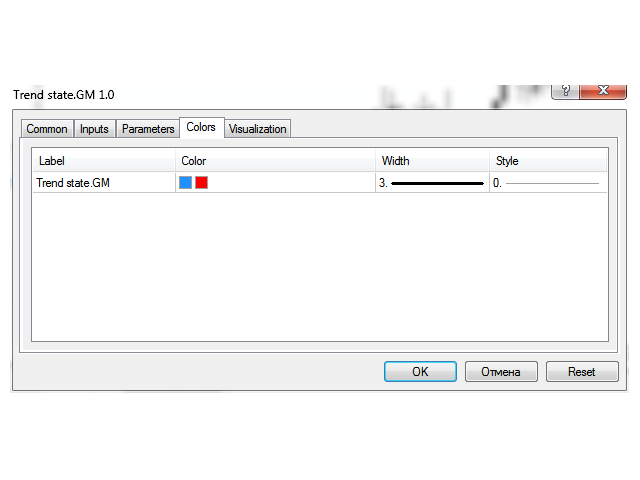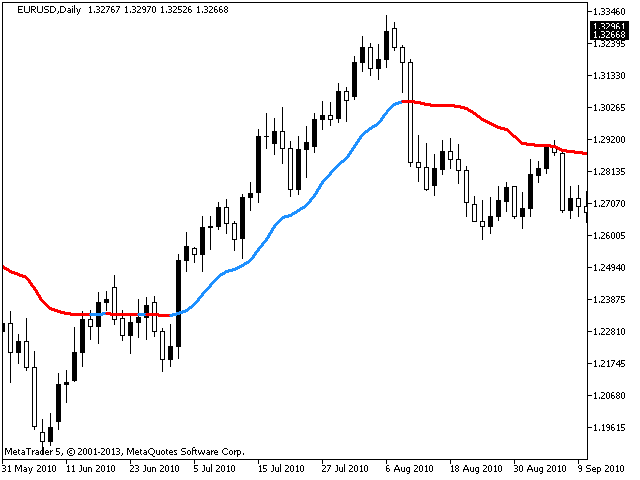### Download source code of Trend state Geometrical Mean for only 97 usd